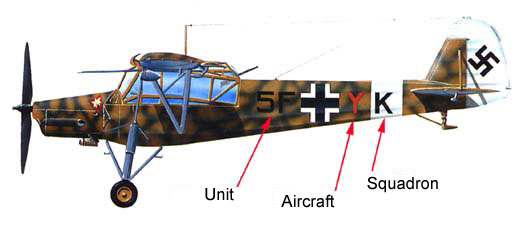# Alpha codes of combat wings (units) and squadronsAlpha codes of combat wings (units) and squadrons

Buchstabenschlüssel der Kampfverbände der Luftwaff

Πηγή: www.rlm.atThe alpha codes of fighting units
Standard and exceptions considering the number of groups:
 A = wing staff (a blue letter to the left) B = staff of the 1st group (a green letter to the left) C = staff of the 2nd group (a green letter to the left) D = staff of the 3rd group (a green letter to the left) E = staff of the 4th group (a green letter to the left) F = staff of the 5th group (a green letter to the left)
 1st Group H = 1st Squadron (a white letter to the left) K = 2nd Squadron (a red letter to the left) L = 3rd Squadron (a yellow letter to the left)
 2nd Group M = 4th Squadron (a white letter to the left) N = 5th Squadron (a red letter to the left) P = 6th Squadron (a yellow letter to the left)
 3rd Group R = 7th Squadron (a white letter to the left) S = 8th Squadron (a red letter to the left) T = 9th Squadron (a yellow letter to the left)
 4th Group U = 10th Squadron (a white letter to the left) V = 11th Squadron (a red letter to the left) W = 12th Squadron (a yellow letter to the left)
 5th Group X = 13th Squadron (a white letter to the left) Y = 14th Squadron (a red letter to the left) Z = 15th Squadron (a yellow letter to the left)